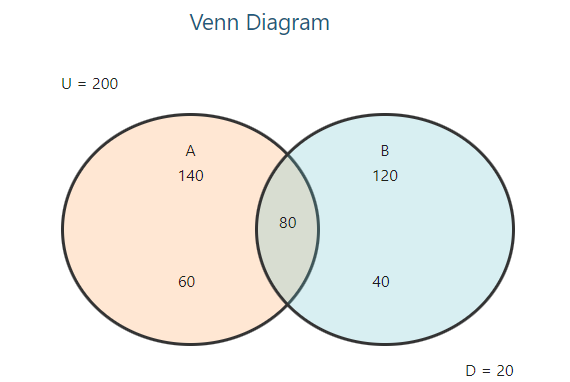•Sign In
• Blog
• Write for UsUh Oh! It seems you’re using an Ad blocker!

We always struggled to serve you with the best online calculations, thus, there's a humble request to either disable the AD blocker or go with premium plans to use the AD-Free version for calculators.

Or# Venn Diagram Calculator

Enter all the required values in their respective fields and the calculator will draw a venn diagram, with steps shown.

Venn Diagram for Two Sets
Venn Diagram for Three Sets

Enter the title of the venn diagram:

Enter the title of A :

Enter the title of B :

Enter the value of A:

Enter the value of B:

Enter the value of Universal(U) :

Enter the value of A∩B:

Enter the title of the venn diagram:

Enter the title of A :

Enter the title of B :

Enter the title of C :

Enter the value of A:

Enter the value of B:

Enter the value of C :

Enter the value of Universal(U) :

Enter the value of A∩B:

Enter the value of B∩C :

Enter the value of C∩A :

Enter the value of A∩B∩C :

Table of Content

Get the Widget!

Add this calculator to your site and lets users to perform easy calculations.

Feedback

How easy was it to use our calculator? Did you face any problem, tell us!

The probability Venn diagram calculator generates the symmetric Venn diagram of two or three sets of data. Not only this, but you can also get union and intersection results for given values by using this Venn diagram solver.

## What Is a Venn Diagram?

The Venn diagram is a basic representation of all the similarities and differences of the set of data.

The other name of the Venn diagram is the logic diagram and it represents all the possible logical possibilities or probabilities. In set mathematics, it is the most efficient model to represent the relationship between the sets of data by a simple graphical representation.

The similarities are the intersection values and the differences are the unions of dataset values. You may not get to know how to figure out union and intersection numbers in data set groups. But do not worry as our Venn diagram calculator will do it for you in seconds.

## Venn Diagram Formula:

n (A ∪B) = n (A) + n(B) – n (A ∩ B)

n ( A∪ B ∪ C) = n(A) + n(B) + n(C) – n( B∩ Y) – n( B ∩ C) – n ( C ∩ XA) + n( A  ∩ B ∩ C)

Our Venn diagram probability calculator also makes use of the above-mentioned formulas to generate accurate outcomes in a glimpse of moments.

## Example:

In a university, 200 students are randomly selected. Now 140 students like football, 120 like cricket, and 80 like both football and cricket.

How many students only like football?

How many students only like cricket?

How many students like both football and cricket?

How many students like neither football nor cricket?

### Solution:

Here set A represents the students who like football set B the students who like cricket, and the intersection of A∩B, the students who like both football and cricket.The 3 circle Venn diagram template elaborates that the students only like football or cricket with the students like both the game.

The Students Like Only the Football:

A-only = A – A∩B

A-only = 140-80

A only = 60

The Students Like Only the Cricket:

B-only = B – A∩B

B-only = 120-80

B-only = 40

Students Like Both Football and Cricket

A= 140

B = 120

A∩B = 80

Students Like Neither Football Nor Cricket:

D = U – [A only + B only + (A∩B)]

D = 200 – [(60) + (40) + 80]

D = 20

The 3 Venn diagram picture also represents the students neither like cricket nor football.

## Working of Venn Diagram Calculator:

Our probability Venn diagram solver requires a few values to calculate the results. These include:

Input:

• Select the Venn diagram for two or three sets
• Enter the values of the set A, B, A∩B, and the universal set
• Hit the calculate the Button

Output:

• Venn diagram of sets
• Step-by-step calculations

## FAQs

### What Is a Venn Diagram With 3 Circles Called?

The 3 circle Venn diagram template is called the triple Venn diagram. The triple Venn diagram maker is used to compare three categories. The overlapping region represents the shared region between the three categories.

### What Are the HCF and LCM In a Venn Diagram?

The intersection is the HCF (highest common factor) and the union is the LCM (Least common multiple) in the Venn diagram. The Venn diagram calculator calculates both the LCM and HCF of the 2 and 3 sets.

## References:

From the source of Wikipedia: Venn diagram 3 Circles, Detail

From the source of investopedia.com: Venn Diagram Component, 3 Part Venn Diagram